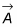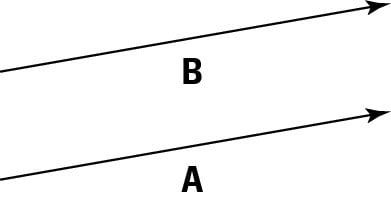##### Physics I For DummiesIn physics, when you have a vector, you have to keep in mind two quantities: its direction and its magnitude. Quantities that have only a magnitude are called scalars. If you give a scalar magnitude a direction, you create a vector.

Visually, you see vectors drawn as arrows, which is perfect because an arrow has both a clear direction and a clear magnitude (the length of the arrow). Take a look at the following figure. The arrow represents a vector that starts at the arrow’s foot (also called the tail) and ends at the head.A vector, represented by an arrow, has both a direction and a magnitude.

In physics, you generally use a letter in bold type to represent a vector, although you may also see a letter with an arrow on top like this:The arrow means that this is not only a scalar value, which would be represented by A, but also something with direction.

Say that you tell some smartypants that you know all about vectors. When he asks you to give him a vector, A, you give him not only its magnitude but also its direction, because you need these two bits of info together to define this vector. That impresses him to no end! For example, you may say that A is a vector at 15° from the horizontal with a magnitude of 12 meters/second. Smartypants knows all he needs to know, including that A is a velocity vector.Equal vectors have the same length and direction but may have different starting points.

Take a look at this figure, which features two vectors, A and B. They look pretty much the same — the same length and the same direction. In fact, these vectors are equal. Two vectors are equal if they have the same magnitude and direction, and you can write this as A = B.